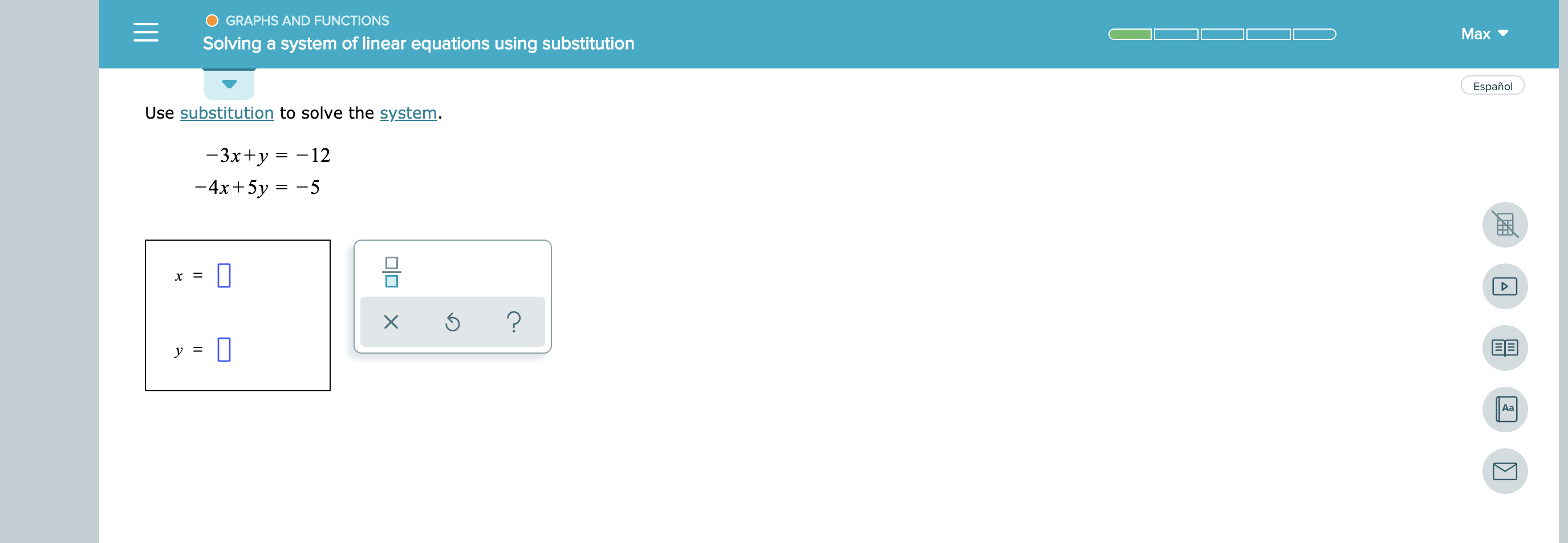O GRAPHS AND FUNCTIONSMaxSolving a system of linear equations using substitutionEspañolUse substitution to solve the system.— 3x+у 3D -12-4x+5y 5х 3?EEуАаX

Question

see attachmenthelp_outlineImage TranscriptioncloseO GRAPHS AND FUNCTIONS Max Solving a system of linear equations using substitution Español Use substitution to solve the system. — 3x+у 3D -12 -4x+5y 5 х 3 ? EE у Аа X fullscreen
Step 1

From the first equation: -3x + y = -12

Hence, y = 3x - 12

Let's substitute this in the second equation.

Step 2

-4x + 5y = - 5

Or, -4x + 5(3x - 12) = - 5

Or, 11x - 60 = -5

Hence, x = (60 - 5) / 11 = 5

Step 3

And y = 3x - 12 = 3 ...

Want to see the full answer?

See Solution

Want to see this answer and more?

Our solutions are written by experts, many with advanced degrees, and available 24/7

See Solution
Tagged in

Calculus